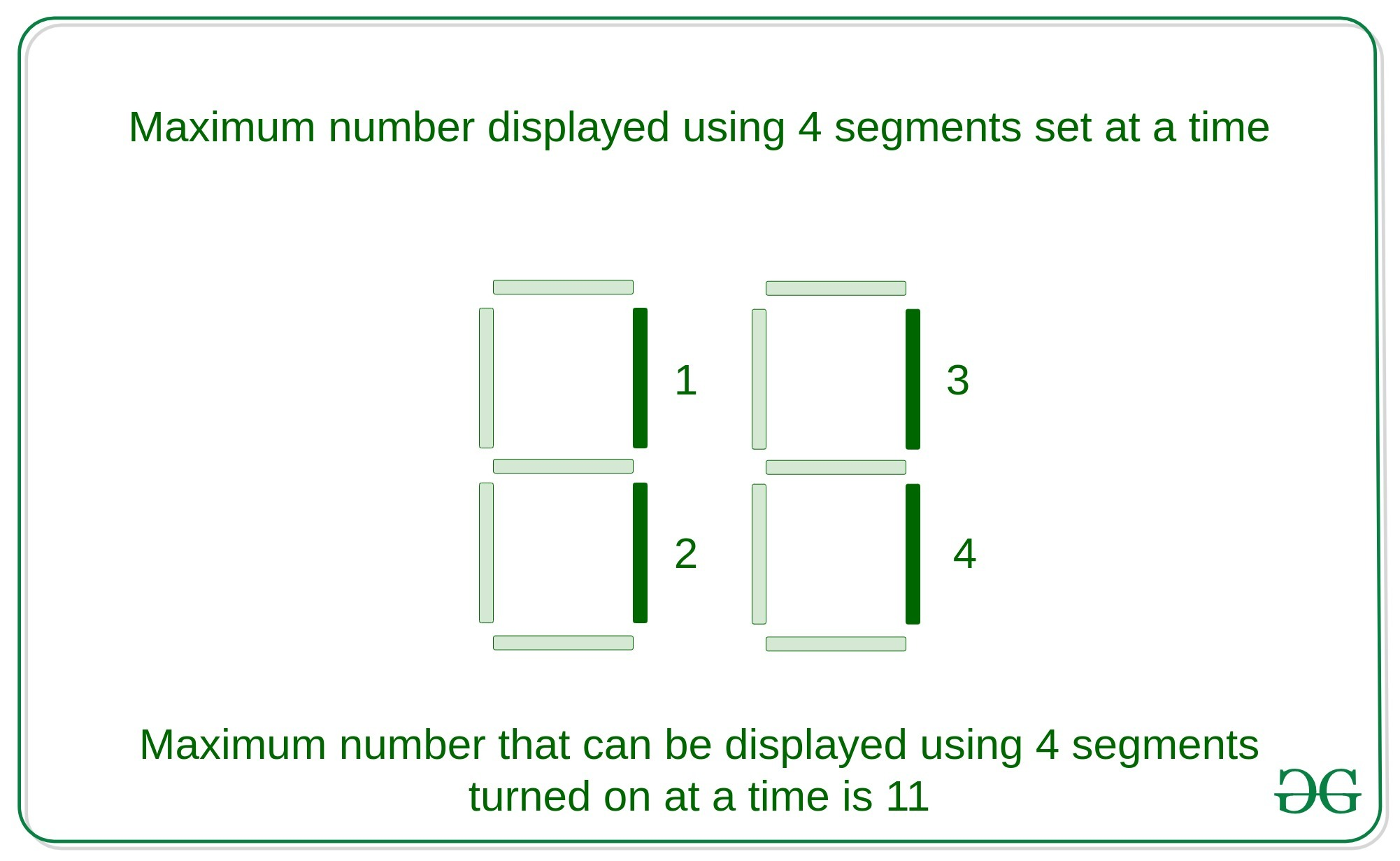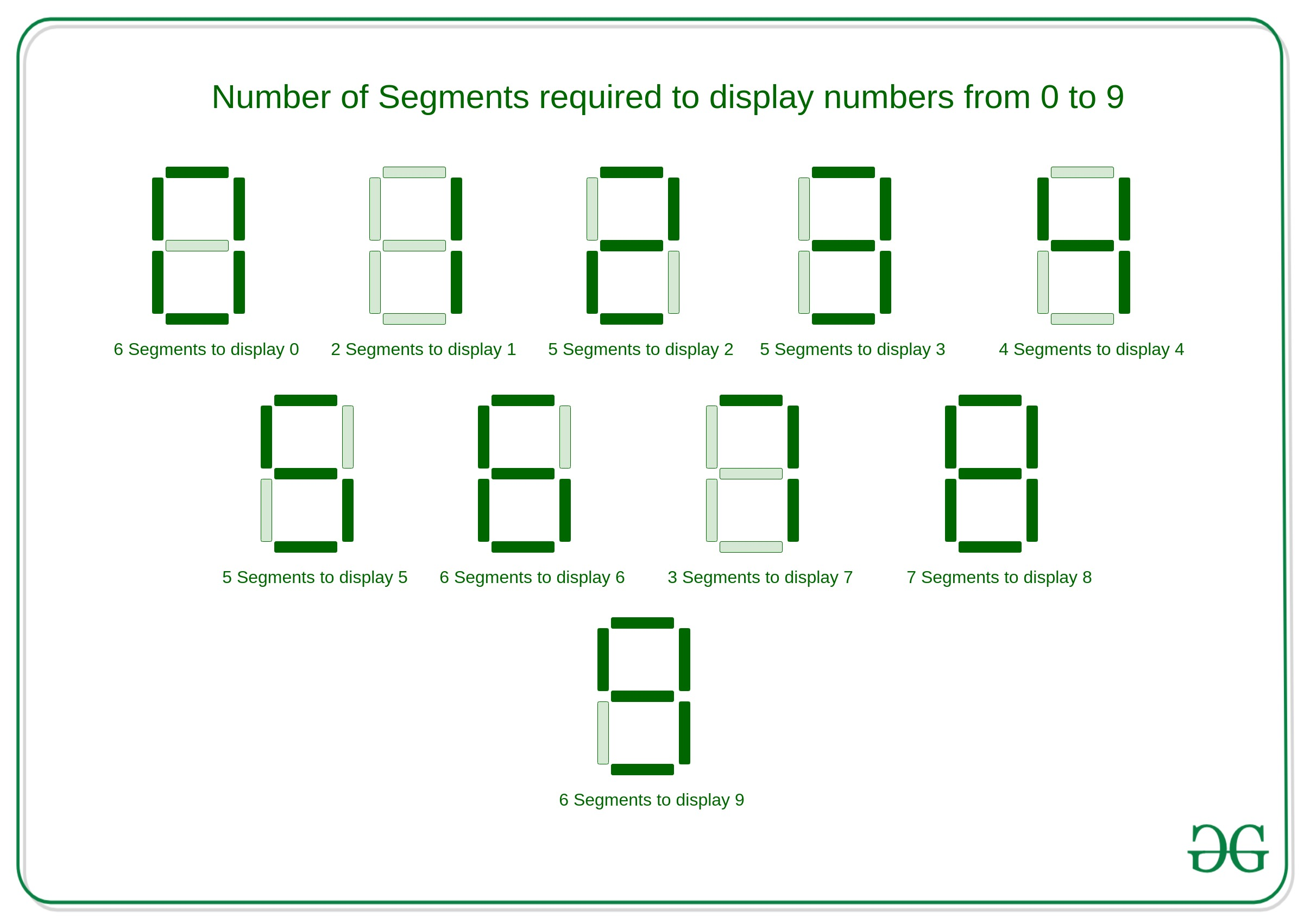# Maximum number on 7-segment display using N segments : Recursive

Given an integer N, the task is to find the largest number that can be shown with the help of N segments using any number of 7 segment displays.

Examples:

Input: N = 4
Output: 11
Explanation:
Largest number that can be displayed with the help of 4 segments using 2 seven segment displays turned is 11.Input: N = 7
Output: 711
Explanation:
Largest number that can be displayed by turning on seven segments is 711 with the help of 3 segments display set.

## Recommended: Please try your approach on {IDE} first, before moving on to the solution.

Approach:
The key observation in seven segment display is to turn on any number from 0 to 9 takes certain amounts of segments, which is described below:If the problem is observed carefully, then the number N can be of two types that is even or odd and each of them should be solved separately as follows:

• For Even: As in the above image, There are 6 numbers that can be displayed using even number of segments which is
```0 - 6
1 - 2
2 - 5
4 - 4
6 - 6
9 - 6
```

As it is observed number 1 uses the minimum count of segments to display a digit. Then, even the number of segments can be displayed using 1 with 2 counts of segments in each digit.

• For Odd: As in the above image, there are 5 numbers that can be displayed using an odd number of segments which is
```3 - 5
5 - 5
7 - 3
8 - 7
```

As it is observed number 7 uses the minimum number of odd segments to display a digit. Then an odd number of segments can be displayed using 7 with 3 counts of segments in each digit.

Algorithm:

• If the given number N is 0 or 1, then any number cannot be displayed with this much of bits.
• If the given number N is odd then the most significant digit will be 7 and the rest of the digits can be displayed with the help of the (N – 3) segments because to display 7 it takes 3 segments.
• If given number N is even then the most significant digit will be 1 and the rest of the digits can be displayed with the help of the (N – 2) segments because to display 1, it takes 2 segments only.
• The number N is processed digit by digit recursively.

Explanation with Example:
Given number N be – 11

Digit (from MSB to LSB) N Largest number from N using segment Segments used Segments remaining
1 11 7 3 8
2 8 1 2 6
3 6 1 2 4
4 4 1 2 2
5 2 1 2 0

Then, the largest number will be 71111.

Below is the implementation of the above approach:

## C++

 `// C++ implementation to find the ` `// maximum number that can be  ` `// using the N segments in  ` `// N segments display ` ` `  `#include ` `using` `namespace` `std; ` ` `  `// Function to find the maximum ` `// number that can be displayed ` `// using the N segments  ` `void` `segments(``int` `n) ` `{ ` `    ``// Condition to check base case ` `    ``if` `(n == 1 || n == 0) { ` `        ``return``; ` `    ``} ` `    ``// Condition to check if the  ` `    ``// number is even ` `    ``if` `(n % 2 == 0) { ` `        ``cout << ``"1"``; ` `        ``segments(n - 2); ` `    ``} ` `     `  `    ``// Condition to check if the ` `    ``// number is odd ` `    ``else` `if` `(n % 2 == 1) { ` ` `  `        ``cout << ``"7"``; ` `        ``segments(n - 3); ` `    ``} ` `} ` ` `  `// Driver Code ` `int` `main() ` `{ ` `    ``int` `n; ` `    ``n = 11; ` `    ``segments(n); ` `    ``return` `0; ` `} `

## Java

 `// Java implementation to find the  ` `// maximum number that can be  ` `// using the N segments in  ` `// N segments display  ` `class` `GFG { ` `     `  `    ``// Function to find the maximum  ` `    ``// number that can be displayed  ` `    ``// using the N segments  ` `    ``static` `void` `segments(``int` `n)  ` `    ``{  ` `        ``// Condition to check base case  ` `        ``if` `(n == ``1` `|| n == ``0``) {  ` `            ``return``;  ` `        ``}  ` `        ``// Condition to check if the  ` `        ``// number is even  ` `        ``if` `(n % ``2` `== ``0``) {  ` `            ``System.out.print(``"1"``);  ` `            ``segments(n - ``2``);  ` `        ``}  ` `         `  `        ``// Condition to check if the  ` `        ``// number is odd  ` `        ``else` `if` `(n % ``2` `== ``1``) {  ` `     `  `            ``System.out.print(``"7"``);  ` `            ``segments(n - ``3``);  ` `        ``}  ` `    ``}  ` `     `  `    ``// Driver Code  ` `    ``public` `static` `void` `main (String[] args) ` `    ``{  ` `        ``int` `n;  ` `        ``n = ``11``;  ` `        ``segments(n);  ` `    ``}  ` `} ` ` `  `// This code is contributed by AnkitRai01 `

## Python3

 `# Python3 implementation to find the  ` `# maximum number that can be  ` `# using the N segments in  ` `# N segments display  ` ` `  `# Function to find the maximum  ` `# number that can be displayed  ` `# using the N segments  ` `def` `segments(n) : ` ` `  `    ``# Condition to check base case  ` `    ``if` `(n ``=``=` `1` `or` `n ``=``=` `0``) : ` `        ``return``;  ` `     `  `    ``# Condition to check if the  ` `    ``# number is even  ` `    ``if` `(n ``%` `2` `=``=` `0``) : ` `        ``print``(``"1"``,end``=``"");  ` `        ``segments(n ``-` `2``);  ` `     `  `    ``# Condition to check if the  ` `    ``# number is odd  ` `    ``elif` `(n ``%` `2` `=``=` `1``) : ` ` `  `        ``print``(``"7"``,end``=``"");  ` `        ``segments(n ``-` `3``);  ` ` `  `# Driver Code  ` `if` `__name__ ``=``=` `"__main__"` `:  ` ` `  `    ``n ``=` `11``;  ` `    ``segments(n);  ` ` `  `# This code is contributed by AnkitRai01 `

## C#

 `// C# implementation to find the  ` `// maximum number that can be  ` `// using the N segments in  ` `// N segments display  ` `using` `System; ` ` `  `class` `GFG { ` `     `  `    ``// Function to find the maximum  ` `    ``// number that can be displayed  ` `    ``// using the N segments  ` `    ``static` `void` `segments(``int` `n)  ` `    ``{  ` `        ``// Condition to check base case  ` `        ``if` `(n == 1 || n == 0) {  ` `            ``return``;  ` `        ``}  ` `        ``// Condition to check if the  ` `        ``// number is even  ` `        ``if` `(n % 2 == 0) {  ` `            ``Console.Write(``"1"``);  ` `            ``segments(n - 2);  ` `        ``}  ` `         `  `        ``// Condition to check if the  ` `        ``// number is odd  ` `        ``else` `if` `(n % 2 == 1) {  ` `     `  `            ``Console.Write(``"7"``);  ` `            ``segments(n - 3);  ` `        ``}  ` `    ``}  ` `     `  `    ``// Driver Code  ` `    ``public` `static` `void` `Main() ` `    ``{  ` `        ``int` `n;  ` `        ``n = 11;  ` `        ``segments(n);  ` `    ``}  ` `} ` ` `  `// This code is contributed by AnkitRai01 `

Output:

```71111
```

Performance Analysis:

• Time Complexity: As in the above approach, there is recursive call which takes O(N) time in worst case, Hence the Time Complexity will be O(N).
• Auxiliary Space Complexity: As in the above approach, taking consideration of the stack space used in recursive call then the auxiliary space complexity will be O(N)

Attention reader! Don’t stop learning now. Get hold of all the important DSA concepts with the DSA Self Paced Course at a student-friendly price and become industry ready.

My Personal Notes arrow_drop_upCheck out this Author's contributed articles.

If you like GeeksforGeeks and would like to contribute, you can also write an article using contribute.geeksforgeeks.org or mail your article to contribute@geeksforgeeks.org. See your article appearing on the GeeksforGeeks main page and help other Geeks.

Please Improve this article if you find anything incorrect by clicking on the "Improve Article" button below.

Improved By : AnkitRai01, Akanksha_Rai

Article Tags :
Practice Tags :

Be the First to upvote.

Please write to us at contribute@geeksforgeeks.org to report any issue with the above content.